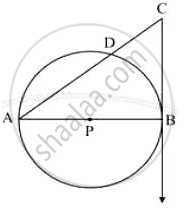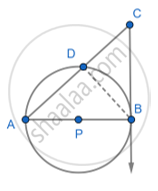# In the Following Figure, Seg Ab is the Diameter of the Circle with Center P. Line Cb Be the Tangent and Line Ac Intersects a Circle in Point D. Prove That: Ac X Ad = 4 (Radius)2 - Geometry

Sum

In the following figure, seg AB is the diameter of the circle with center P. Line CB be the tangent and line AC intersects a circle in point D. Prove that:#### Solution

Given: A circle with center P. CB tangent and line AC intersect a circle in point D

Construction: Join BD.To Prove:  ADB =90° [Angle inscribed in semicircle]

PBC = 90° [Tangent perpendicular to the radius]

i.e. ABC =90°

In Δ ACB and Δ ABD

ABC =  ADB [Each is of 90°]

CAB = DAB [Common angle]

ΔACB  ΔABD [AA property]

"AC"/"AB"="AB"/"AD"

AP = PB …(radii of the same circle)

AB = AP +PB

AB = 2AP

Substituting the value of AB in equation (1)

Concept: Tangent to a Circle
Is there an error in this question or solution?